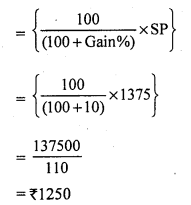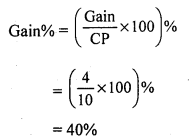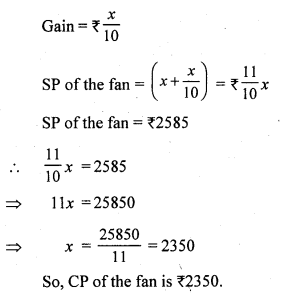# RS Aggarwal Class 7 Solutions Chapter 11 Profit and Loss CCE Test Paper

In this chapter, we provide RS Aggarwal Solutions for Class 7 Chapter 11 Profit and Loss CCE Test Paper for English medium students, Which will very helpful for every student in their exams. Students can download the latest RS Aggarwal Solutions for Class 7 Chapter 11 Profit and Loss CCE Test Paper Maths pdf, free RS Aggarwal Solutions Class 7 Chapter 11 Profit and Loss CCE Test Paper Maths book pdf download. Now you will get step by step solution to each question.

### RS Aggarwal Solutions for Class 7 Chapter 11 Profit and Loss CCE Test Paper Download PDF

Question 1.
Solution:
SP of the chair = ₹ 1375
Gain% = 10
CP of the chairQuestion 2.
Solution:
Let the cost of each pen be ₹ 1
CP of 10 pens = ₹ 10
SP of 10 pens = CP of 14 pens = ₹ 14
Gain = SP – CP = 14 – 10 = ₹ 4Question 3.
Solution:
Let the cost price of the fan be ₹ xQuestion 4.
Solution:
Cost price of six lemons = ₹ 10Question 5.
Solution:
SP of the bat = ₹ 486
Loss = 10%
CP of the batMark (✓) against the correct answer in each of the following :
Question 6.
Solution:
(b) ₹ 85
SP of a football = ₹ 100
Gain = ₹ 15
Gain = SP – CP
⇒ 15 = 100 – CP
⇒ CP = ₹ (100 – 15)
⇒ CP = ₹ 85

Question 7.
Solution:
(c) 15.2%
Cost price of 12 bananas = ₹ 25
Cost price of one banana = ₹ 2512
Cost price of five bananas = 5 x 2512
= 12512 = 10.42
He shells five bananas at the cost (SP) of ₹ 12.
Gain = SP – CP = ₹ (12 – 10.42) = ₹ 1.58Question 8.
Solution:
(c) ₹ 196
Let the cost price of the jug be ₹ xQuestion 9.
Solution:
(c) 6623 %
Let the cost price of each banana be ₹ 1
Cost price of three bananas = ₹ 3
SP of three bananas = CP of five bananas = ₹ 5
Gain = SP – CP = ₹ (5 – 3) = ₹ 2Question 10.
Solution:
(i) Loss = (CP) – (SP).Question 11.
Solution:
(i) False
Gain or loss is always reckoned on the cost price.
(ii) True
(iii) True
(iv) True

All Chapter RS Aggarwal Solutions For Class 7 Maths

—————————————————————————–

All Subject NCERT Exemplar Problems Solutions For Class 7

All Subject NCERT Solutions For Class 7

*************************************************

I think you got complete solutions for this chapter. If You have any queries regarding this chapter, please comment on the below section our subject teacher will answer you. We tried our best to give complete solutions so you got good marks in your exam.

If these solutions have helped you, you can also share rsaggarwalsolutions.in to your friends.